Open in App
Not now

## Related Articles

• CBSE Class 12 Syllabus
• CBSE Class 12 Maths Notes
• CBSE Class 12 Physics Notes
• CBSE Class 12 Chemistry Notes
• CBSE Class 12 Accountancy Notes
• CBSE Class 12 Computer Science (Self-Paced Course)

# Continuity and Discontinuity in Calculus – Class 12 CBSE

• Difficulty Level : Expert
• Last Updated : 12 Nov, 2020

A function is said to be continuous if one can sketch its curve on a graph without lifting the pen even once. A function is said to be continuous at x = a, if, and only if the three following conditions are satisfied.

1. The function is defined at x = a; that is, f(a) equals a real number
2. The limit of the function as x approaches a exists
3. The limit of the function as x approaches a is equal to the function value at x = a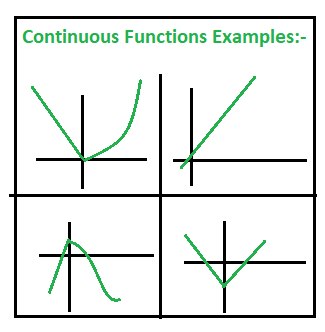A function f(x) is said to be continuous in the open interval (a, b) if at any point in the given interval the function is continuous. In the case of closed interval [a, b], the function is said to be continuous:

• f(x) is be continuous in the open interval (a, b)
• limx⇢a f(x) = f(a)
• limx⇢b f(x) = f(b)

Example 1: Prove that the function f(x) = 5x – 3 is continuous at x = 0.

Solution:

Given, f(x) = 5x – 3

At x = 0 , f(0) = (5 × 0) – 3 = -3
limx⇢0 f(x) = limx⇢0 (5x – 3) = (5 × 0) – 3 = -3
limx⇢0 f(x) = f(0)

Therefore, f(x) is continuous at x = 0.

Example 2: Examine the function f(x) = |x – 5|, for continuity.

Solution:

Given function, f(x) = |x – 5|

Domain of f(x) is real and infinite for all real x
Here , f(x) = |x – 5| is a modulus function
As , every modulus function is continuous
Therefore , f(x) is continuous in its domain R.

Example 3: Is the function f(x) =  x – sinx + 5  is continuous at x = π.

Solution:

Given function is f(x) = x – sinx + 5

L.H.L = limx⇢π (x – sinx + 5) = limx⇢π[(π – h) – sin(π – h) + 5] = π + 5

R.H.L = limx⇢π+ (x – sinx + 5) = limx⇢π+ [(π + h) – sin(π + h) + 5] = π + 5

And, f(π) = π – sinπ + 5 = π  + 5

Since , L.H.L = R.H.L = f(π)
Therefore , f(x) is continuous at x = π

Example 4: Examine the continuity of the function f(x) = 2x – 1 at x = 3.

Solution:

Given f(x) = 2x – 1

At x = 3, f(x) = (2 × 3) – 1 = 5
limx⇢3 f(x) = limx⇢3 f(x) = (2×3) – 1 = 5
limx⇢3 f(x) = f(3)
Therefore, f(x) is continuous at x = 3

Example 5: Examine the function is continuous or not?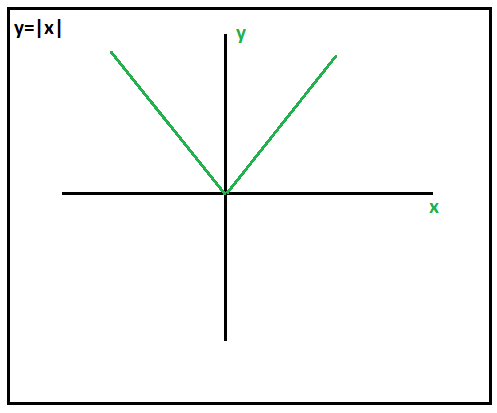Solution:

For x > 0, y = x and x < 0, y = -x

So, We Know it is continuous for x > 0 and x < 0. To check if it is continuous at x = 0 , check the limit:

limx⇢0  |x| = limx⇢0 (-x) = 0

limx⇢0+  |x| = limx⇢0+ (x) = 0

So, limx⇢0 |x| = 0 , which is equal to the value of the function at 0. Therefore, It is continuous everywhere.

## Discontinuity

A function is discontinuous at a point x = a if the function is not continuous at a. The function “f” is said to be discontinuous at x = a in any of the following cases:

1. f(a) is not defined
2. limx⇢a+ f(x) and limx⇢a–  f(x) exists, but are not equal.
3. limx⇢a+ f(x) and limx⇢a f(x) exists and are equal but not equal to f(a).

### Types of Discontinuities

There are three basic types of discontinuities

1. Removable(point) Discontinuity
2. Infinite Discontinuity
3. Jump Discontinuity

Removable(point) Discontinuity: The graph has a hole at a single x-value. Imagine you’re walking down the road, and someone has removed a manhole cover. This is a category of discontinuity in which the function has a well defined two-sided limit at x = a, but either f(a) is not defined or f(a) is not equal to its limit.

• limx⇢a f(x) ≠ f(a)
• f(a) = limx⇢a f(x)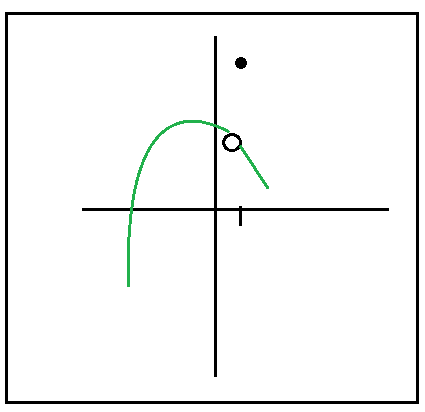Infinite Discontinuity: The function goes toward positive or negative infinity. Imagine a road getting closer and closer to a river with no bridge to the other side. The function diverges at x = a to give it a discontinuous nature here. That is to say, f(a) is not defined. Since the value of the function at x = a tends to infinity or doesn’t approach a particular finite value, the limits of the function as x → a are also not defined.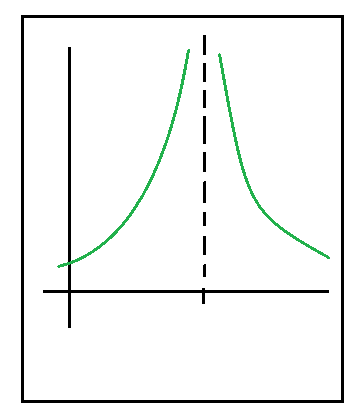Jump Discontinuity: The graph jumps from one place to another. Imagine a superhero going for a walk, he reaches a dead end and, because he can, flies to another road. In this type of discontinuity, the right-hand limit and the left-hand limit for the function at x = a exists; but the two are not equal to each other.

• limx⇢a+  f(x) ≠ limx⇢af(x)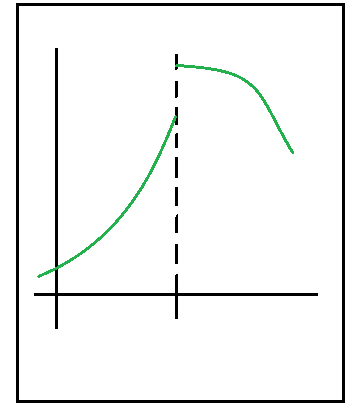My Personal Notes arrow_drop_up